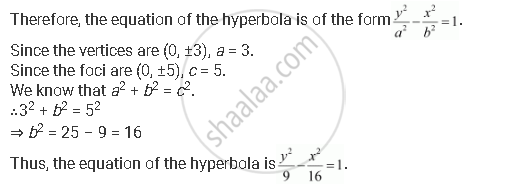CBSE (Arts) Class 11CBSE
Share
Notifications

View all notifications
Books Shortlist
Your shortlist is empty

# Find the Equation of the Hyperbola Satisfying the Give Conditions: Vertices (0, ±3), Foci (0, ±5) - CBSE (Arts) Class 11 - Mathematics

Login
Create free account

Forgot password?
ConceptHyperbola Standard Equation of Hyperbola

#### Question

Find the equation of the hyperbola satisfying the give conditions: Vertices (0, ±3), foci (0, ±5)

#### Solution

Vertices (0, ±3), foci (0, ±5)

Here, the vertices are on the y-axis.Is there an error in this question or solution?

#### APPEARS IN

Solution Find the Equation of the Hyperbola Satisfying the Give Conditions: Vertices (0, ±3), Foci (0, ±5) Concept: Hyperbola - Standard Equation of Hyperbola.
S